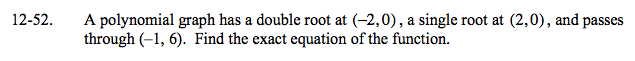### Home > A2C > Chapter 12 > Lesson 12.1.3 > Problem12-52

12-52.

A polynomial graph has a double root at (−2, 0), a single root at (2, 0), and passes through (−1, 6). Find the exact equation of the function. Homework Help ✎Write an equation using the given roots.

y = a(x + 2)2 (x − 2)

Substitute the given point to find the value of a.

y = −2(x + 2)2(x − 2)